# Take A Free ASNT NDT ET Level 3 Exam Practice Test – Quiz Course

Here you can take free ASNT NDT ET Level 3 Practice Test with latest Electromagnetic Testing Method Question and Answers

1.

2.

3.

4.

5.

## In Figure 9, ac flowing through a primary coil set-up a magnetic field and causes a flow of eddy currents in the rod. The voltage of the secondary coil is dependent upon: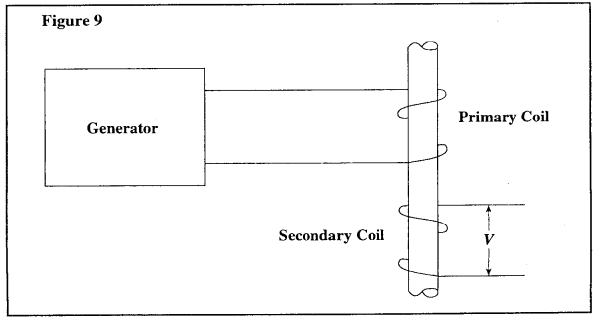6.

7.

8.

9.

10.

11.

12.

13.

14.

15.

16.

17.

18.

19.

20.

21.

22.

23.

24.

25.

26.

27.

28.

29.

30.

31.

## An ac current produces eddy currents in a test object. The vector Hp represents the secondary ac field in the test piece. What function occurs to produce a workable test situation? (See Figure 10.)32.

## To separate cracks and diameter effects for steel cylinders, the optimum frequencies correspond to f/fg ratios of less than (see Figure 11):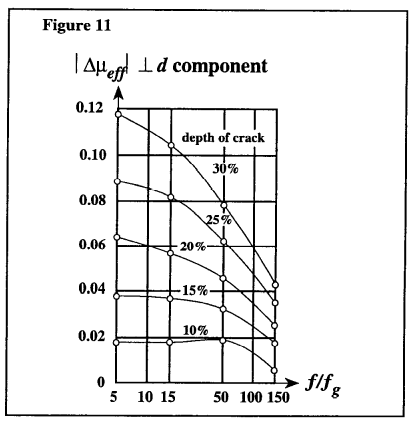33.

## Thin-walled tubes should be tested for cracks, alloy, or wall thickness at frequency ratios between (see Figure 12):34.

## Figure 13 indicates that the largest eddy current indications from subsurface cracks will occur when the frequency ratio (f/fg ) is:35.

## Figure 13 indicates that the magnitude of a signal from a subsurface, the crack will increase when the frequency ratio (f/fg ):36.

## Figure 14 indicates that when inspecting for surface cracks in nonferromagnetic cylinders, the optimum frequency ratio (f/fg ) is between:37.

38.

39.

40.

## Differential coil systems can be of which of the following types? (see Figure 15)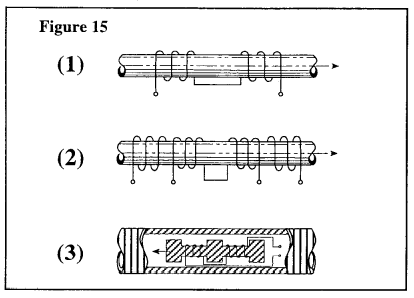41.

42.

43.

44.

45.

46.

47.

48.

49.

## In the ellipse method, the following (see Figure indication should be interpreted as: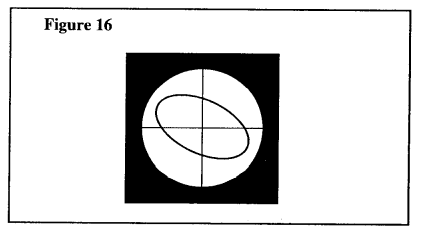50.

51.

52.

53.

54.

55.

56.

57.

58.

59.

60.

61.

62.

63.

64.

65.

66.

67.

68.

69.

70.

71.

72.

73.

74.

75.

## Referring to Figure 17, suppose a test is being conducted at an f/fg, ratio of 1. Due to a mix of alloys, a new test piece with a conductivity value four times as great as the original material is inserted in the coil. The new f/fg, the ratio will be: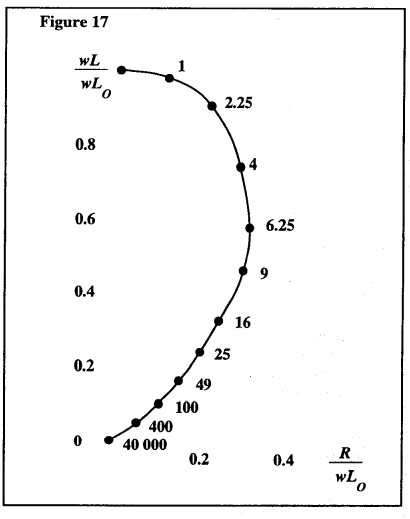76.

## Figure 18 illustrates the separation of diameter effects and conductivity effects: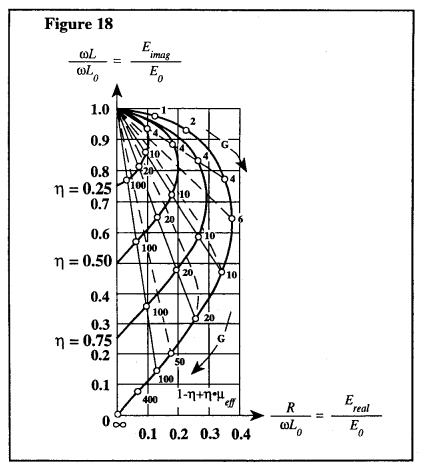77.

## Figure 19 illustrates: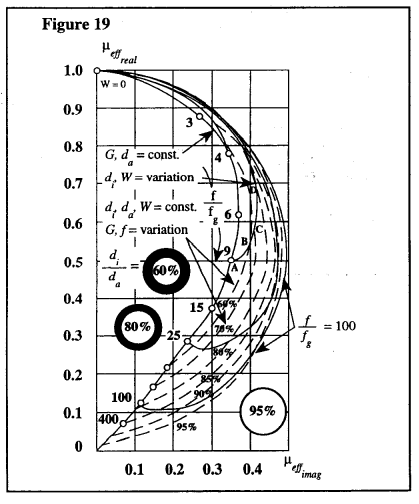78.

## Figure 20 illustrates the impedance diagram for tubing where the ratio of the inside diameter to the outside diameter is 80 percent. It is possible to calculate an approximate limit frequency, fg, by using only the outside diameter. Thus, the maximum test sensitivity for effects of conductivity, wall thickness, and cracks occurs at a frequency ratio of:79.

80.

## In flux leakage testing of wire rope, the system of two different diameter search coils is frequently used. What is the main reason for using such a system? (See Figure FL-8.)81.

## In flux leakage testing of wire rope, two separate outer broken wires will produce two separate indications if there is sufficient distance between the breaks. What is the maximum rope speed in feet per minute that would produce two separate indications when the cut-off frequency of the recorder is 60 kHz and the breaks are 25 mm (1 in.) apart? (See Figure FL-9.)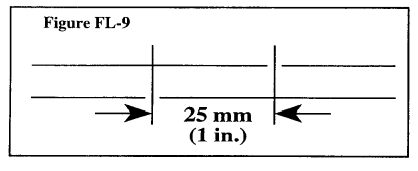82.

83.

## In Figure FL-10, the flux leakage at slot A will be _______than that at slot B.84.

85.

86.

87.

88.

89.

90.

## A defect having an inclined angle to the surface has a flux leakage that is (see Figure FL-11):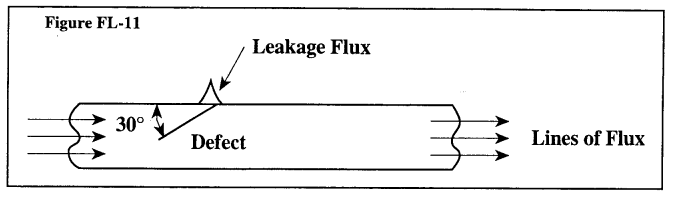91.

92.

## A current-carrying conductor is surrounded by a tube (see Figure FL -12). Magnetic fields exist in the tube if the tube is made of: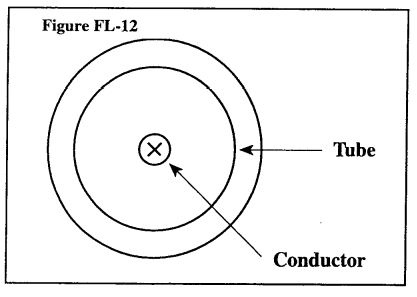93.

## A tube is magnetized by passing a uniform current through the tube. There are inside and outside defects in the tube (see Figure FL-13). The two defects have the same dimension and geometry. The defect signal strength: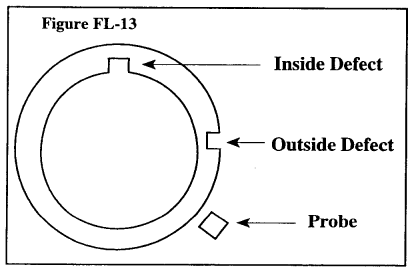94.

95.

96.

97.

98.

99.

## Figure FL-14 shows an arrangement of probe coils in a Forester probe used for measuring:100.

## In flux leakage testing the maximum permissible speed of probe or tube movement with respect to the other is:

Question 1 of 100

## Are You Looking for a Free ASNT NDT ET Level 3Exam Practice Test?

If your answer is yes then you are at the right website. Here You Can Take a Free Online ASNT NDT ET Level 3 Exam Practice Test and Improve Your ASNT ET Level III – Electromagnetic Testing Method Exam Score. Here You Can Learn everything you need to know about ASNT ET Level III quickly and easily.

In This Practice test, We are covering the Latest ASNT ET Level III – Electromagnetic Testing Method Question and Answers, After completing the last Question you will see the ASNT ET Level III Practice Test Answer Key, No cheating now! Once you complete the test and check your answers please leave us your score and any comments in the comments section below

We will help you to pass the ASNT ET Level III Exam on the first attempt —99% Guaranteed. Our expert-written ASNT ET Level III – Electromagnetic Testing Method Examination practice material covers the actual exam topics with fully explained answers.

Take unlimited free ASNT ET Level III Quiz Course tests of any length. We have free Latest questions updated every week and you can study our material along with any other study materials.

In this quiz course, we are covering all the topics listed below:

• Principles of Electromagnetic Testing
• Test Coil Arrangements
• Test Coil Design
• Effects of Test Objects on Test Coils
• Selection of Test Frequency
• Instrument Systems
• Eddy Current Applications
• Alternating Current Field Measurement
• Remote Field Testing
• Electromagnetic Testing Procedures, Specifications, and Standards

Buy ASNT ET LEVEL 3 Exam Learning Package Study Material
FOR EVERY 10 QUESTIONS 1 QUESTION COMES INTO THE EXAM
WE HAVE LATEST ASNT ET Level III – Electromagnetic Testing Method QUESTION BANK
Total 2000+ nos QUESTION BANK FROM THEORY TO PRACTICAL TO NUMERICALS.
Cost: \$25.00